Question

2 long straight wires run parallel to each other, and carry currentS flowing in the same...

2 long straight wires run parallel to each other, and carry currentS flowing in the same direction. Wire 1 carries a current of 1.2A,and wire 2 carries a current of 1.6A. The wires are 15 cm apart.a) What is the magnetic field from wire 1 at the location of wire 2?b)What is the magnetic force per unit length felt by wire 2? C)What is the magnetic force per unit length felt by wire 1?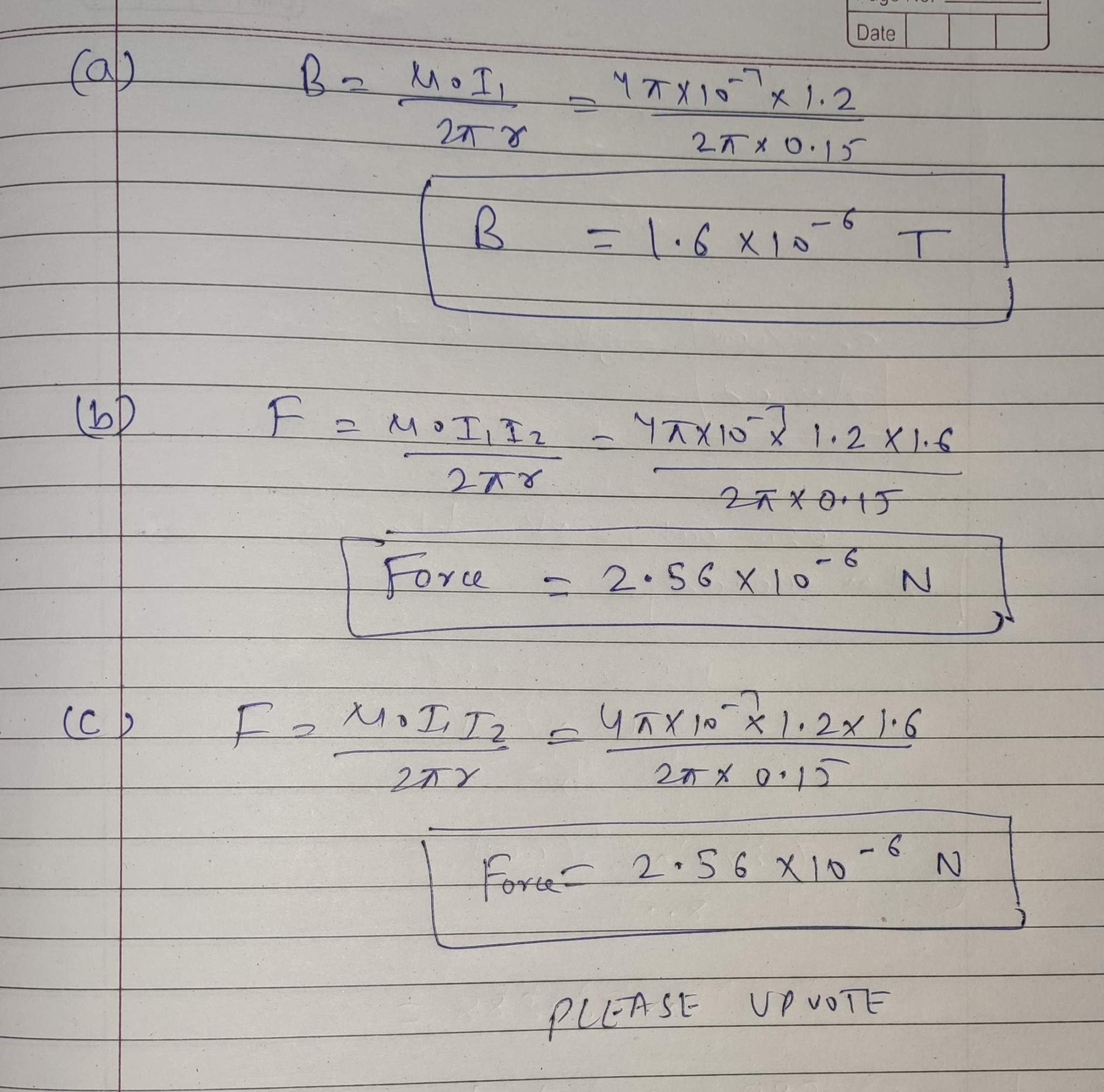Earn Coins

Coins can be redeemed for fabulous gifts.

Similar Homework Help Questions
• 2 long straight wires run parallel to each other, and carry currentS flowing in the same...

2 long straight wires run parallel to each other, and carry currentS flowing in the same direction. Wire 1 carries a current of 1.2A,and wire 2 carries a current of 1.6A. The wires are 15 cm apart.a) What is the magnetic field from wire 1 at the location of wire 2?b)What is the magnetic force per unit length felt by wire 2? C)What is the magnetic force per unit length felt by wire 1?

• Two long straight wires run parallel to each other, and carry currents flowing in the same...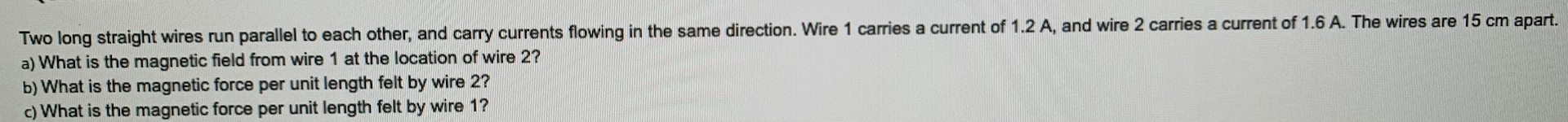Two long straight wires run parallel to each other, and carry currents flowing in the same direction. Wire 1 carries a current of 1.2 A, and wire 2 carries a current of 1.6 A. The wires are 15 cm apart. a) What is the magnetic field from wire 1 at the location of wire 2? b) What is the magnetic force per unit length felt by wire 2? c) What is the magnetic force per unit length felt by wire...

• Problem 1 Three long straight wires are parallel to each other and carry currents directed into...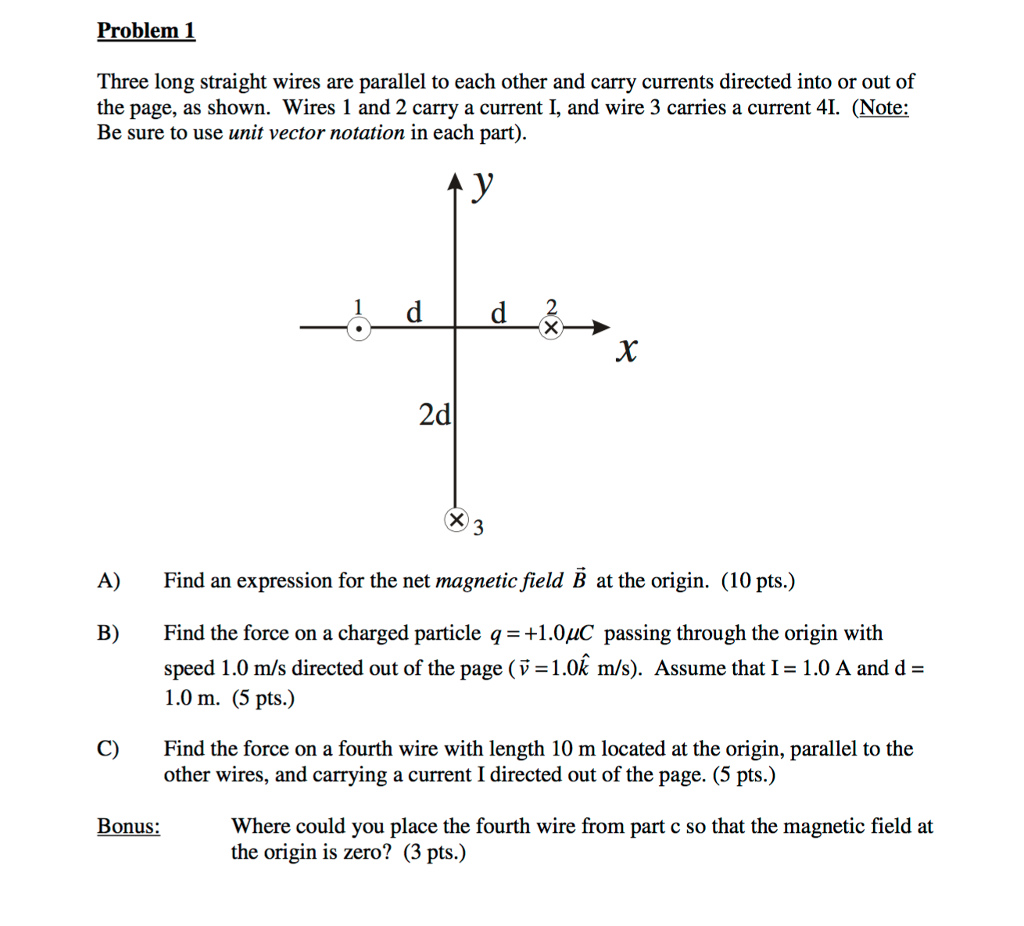Problem 1 Three long straight wires are parallel to each other and carry currents directed into or out of the page, as shown. Wires 1 and 2 carry a current I, and wire 3 carries a current 4. (Note: Be sure to use unit vector notation in each part) 2 2d A) Find an expression for the net magnetic field B at the origin. (10 pts.) B) Find the force on a charged particle q1.0uC passing through the origin with...

• Three long straight wires are parallel to each other and carry currents directed into or out...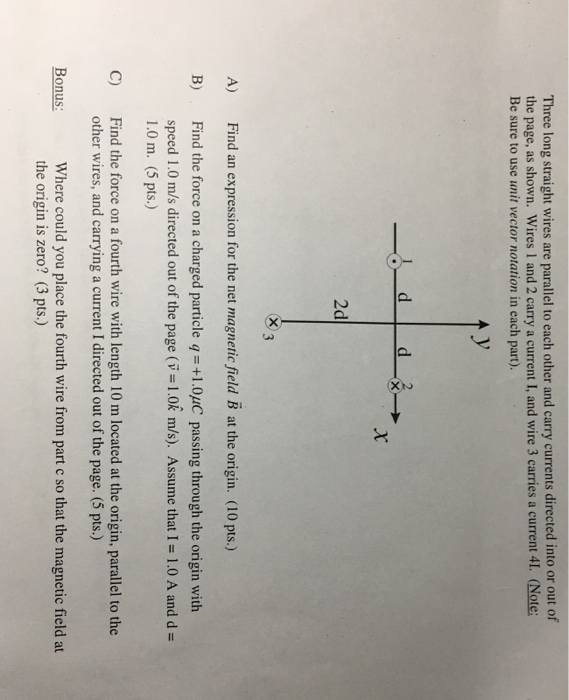Three long straight wires are parallel to each other and carry currents directed into or out of the page, as shown. Wires 1 and 2 carry a current I, and wire 3 carries a current 41. (Note: Be sure to use unit vector notation in each part). 2d indn rssio for th net magnaie fald B ) B) Find the force on a charged particle q-+1.0uC passing through the origin with speed 1.0 m/s directed out of the page (v-1.0k...

• maining Time: 2 hours 08 minutes, 33 seconds estion Completion Status: QUESTION 26 Two long straight...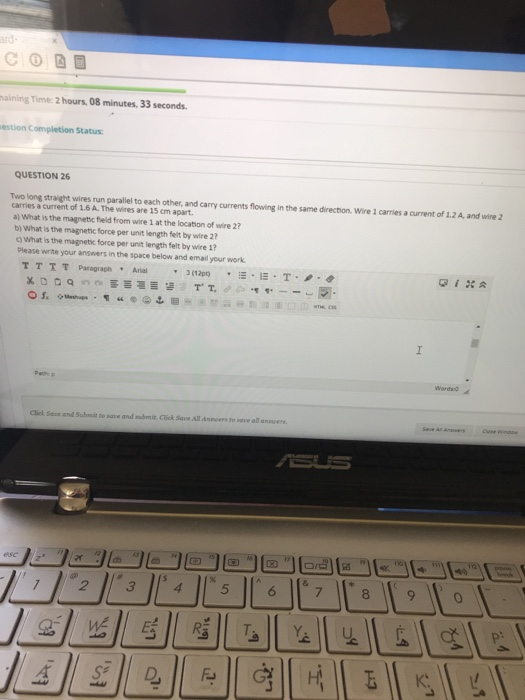maining Time: 2 hours 08 minutes, 33 seconds estion Completion Status: QUESTION 26 Two long straight wires run parallel to each other, and carry currents flowing in the same direction. Wire 1 carries a current of 1.2 A, and wire 2 carries a current of 16 A. The wires are 15 cm apart. a) What is the magnetic field from wire 1 at the location of wire 2? b) What is the magnetic force per unit length felt by wire...

• MULTIPART-3 Two long parallel wires carry currents of 5 A and 7 A in the opposite...

MULTIPART-3 Two long parallel wires carry currents of 5 A and 7 A in the opposite direction. The wires are separated by 0.28 m. a) Find the magnetic force per unit length between the two wires. b) Find the magnetic field midway between the wires c) Find the magnetic force on each wire. The wires are then suspended horizontally one above the other with the currents flowing in same direction. d) If the upper wire is held securely without sagging,...

• Two long, parallel conductors, separated by 13.0 cm, carry currents in the same direction. The first...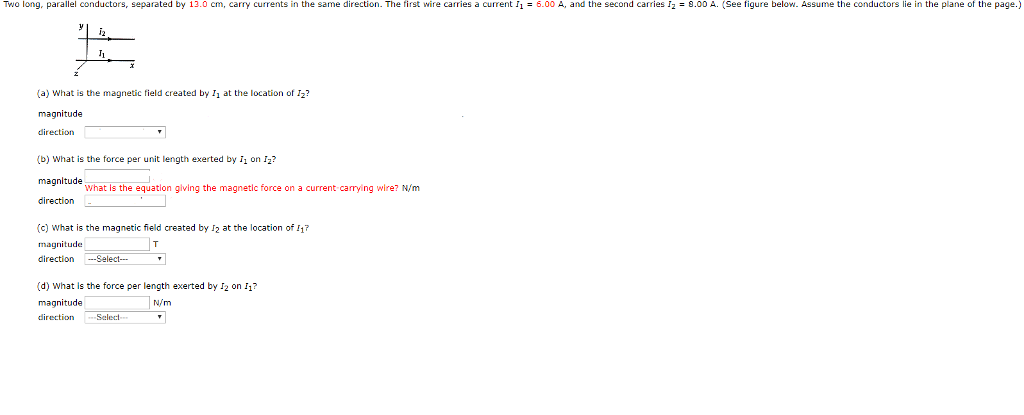Two long, parallel conductors, separated by 13.0 cm, carry currents in the same direction. The first wire carries a current 116.00 A, and the second carries I29.00 A. (See figure below. Assume the conductors lie in the plane of the page.) (a) What is the magnetic field created by I at the location of I2? direction (b) What is the force per unit length exerted by i1 on 12? What Is the equation giving the magnetic force on a current...

• Two long, parallel conductors separated by 10.5 cm carry currents in the same direction. The first...

Two long, parallel conductors separated by 10.5 cm carry currents in the same direction. The first wire carries a current I1 = 3.50 A, and the second carries I2 = 8.00 A. (Assume the conductors lie in the plane of the page.) (a) What is the magnitude of the magnetic field created by I1 at the location of I2? T (b) What is the force per unit length exerted by I1 on I2? magnitude N/m direction (c) What is the...

• 2. The three wires shown in the picture are long and straight, and they each carry the same curre...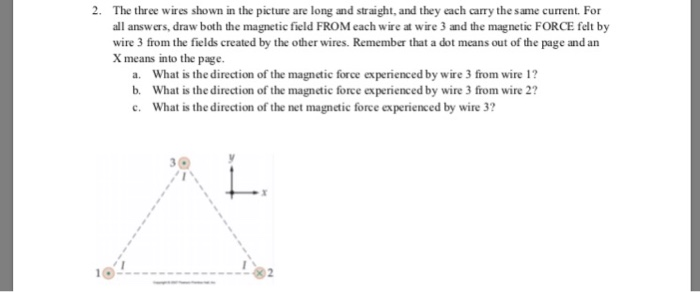please help 2. The three wires shown in the picture are long and straight, and they each carry the same current. For all answers, draw both the magnetic field FROM each wire at wire 3 and the magnetic FORCE felt by wire 3 from the fields created by the other wires. Remember that a dot means out of the page and an X means into the page. a. What is the direction of the magnetic force experienced by wire 3...

• Two long, straight, parallel wires 8.0 cm apart carry currents in opposite directions

Two long, straight, parallel wires 8.0 cm apart carry currents in opposite directions. Use the right-hand source and force rules to determine whether the forces on the wires are attractive or repulsive. a)Repulsive b)Attractive If the wires carry equal currents of 4.0 A, what is the magnetic field magnitude that each produces at the other's location? B= microT Use the result of part C to determine the magnitude of the force per unit length they exert on each other. F/L...